## Addressing Modes in Computer Architecture-

Before you go through this article, make sure that you have gone through the previous article on Addressing Modes.

We have discussed-

• Addressing modes are the different ways of specifying the location of an operand.
• Various kinds of addressing modes and their applications.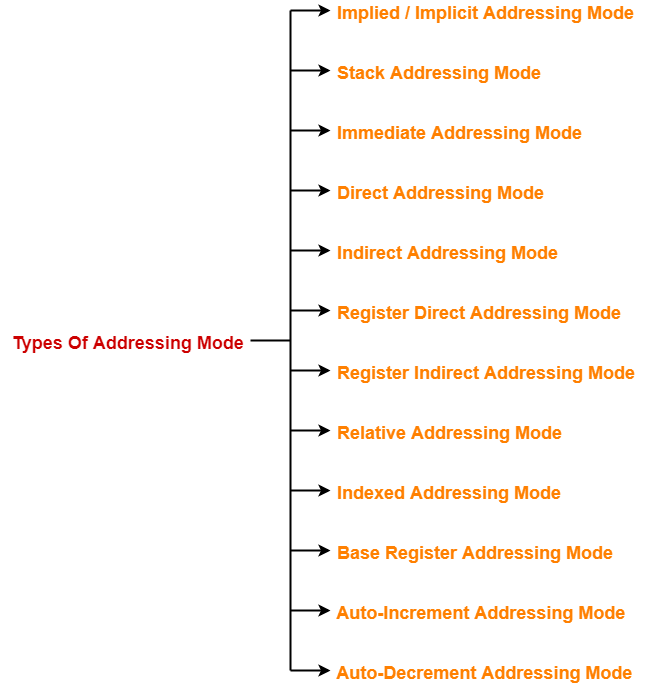## Problem-01:

The most appropriate matching for the following pairs is-

#### Column-2:

1. Loops

2. Pointers

3. Constants

1. X-3, Y-2, Z-1
2. X-1, Y-3, Z-2
3. X-2, Y-3, Z-1
4. X-3, Y-1, Z-2

## Solution-

Option (C) is correct.

## Problem-02:

1. The operand is inside the instruction
2. The address of the operand is inside the instruction
3. The register containing the address of the operand is specified inside the instruction
4. The location of the operand is implicit

## Solution-

Option (B) is correct.

## Problem-03:

Which of the following addressing modes are suitable for program relocation at run time?

1. 1 and 4
2. 1 and 2
3. 2 and 3
4. 1, 2 and 4

## Solution-

Option (C) is correct.

## Problem-04:

What is the most appropriate match for the items in the first column with the items in the second column-

#### Column-2:

1. Array implementation

2. Writing relocatable code

3. Passing array as parameter

1. X-3, Y-1, Z-2
2. X-2, Y-3, Z-1
3. X-3, Y-2, Z-1
4. X-1, Y-3, Z-2

## Solution-

Option (A) is correct.

## Problem-05:

Which of the following addressing modes permits relocation without any change whatsoever in the code?

## Solution-

Option (C) is correct.

## Problem-06:

Consider a three word machine instruction-

The first operand (destination) “A[R0]” uses indexed addressing mode with R0 as the index register. The second operand operand (source) “@B” uses indirect addressing mode. A and B are memory addresses residing at the second and the third words, respectively. The first word of the instruction specifies the opcode, the index register designation and the source and destination addressing modes. During execution of ADD instruction, the two operands are added and stored in the destination (first operand).

The number of memory cycles needed during the execution cycle of the instruction is-

1. 3
2. 4
3. 5
4. 6

## Solution-

For the first operand,

• It uses indexed addressing mode.
• Thus, one memory cycle will be needed to fetch the operand.

For the second operand,

• It uses indirect addressing mode.
• Thus, two memory cycles will be needed to fetch the operand.

After fetching the two operands,

• The operands will be added and result is stored back in the memory.
• Thus, one memory cycle will be needed to store the result.

Total number of memory cycles needed

=  1 + 2 + 1

= 4

Thus, Option (B) is correct.

## Problem-07:

Consider a hypothetical processor with an instruction of type LW R1, 20(R2), which during execution reads a 32-bit word from memory and stores it in a 32-bit register R1. The effective address of the memory location is obtained by the addition of a constant 20 and the contents of register R2. Which of the following best reflects the addressing mode implemented by this instruction for operand in memory?

## Solution-

Clearly, the instruction uses base indexed addressing mode.

Thus, Option (D) is correct.

## Problem-08:

The memory locations 1000, 1001 and 1020 have data values 18, 1 and 16 respectively before the following program is executed.

Which of the statements below is TRUE after the program is executed?

1. Memory location 1000 has value 20
2. Memory location 1020 has value 20
3. Memory location 1021 has value 20
4. Memory location 1001 has value 20

## Solution-

Before the execution of program, the memory is-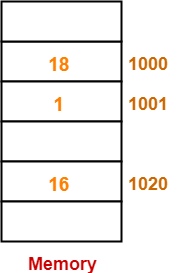Now, let us execute the program instructions one by one-

### Instruction-01: MOVI Rs, 1

• This instruction uses immediate addressing mode.
• The instruction is interpreted as Rs ← 1.
• Thus, value = 1 is moved to the register Rs.

• This instruction uses displacement addressing mode.
• The instruction is interpreted as Rd ← [1000 + [Rs]].
• Value of the operand = [1000 + [Rs]] = [1000 + 1] =  = 1.
• Thus, value = 1 is moved to the register Rd.

• This instruction uses immediate addressing mode.
• The instruction is interpreted as Rd ← [Rd] + 1000.
• Value of the operand = [Rd] + 1000 = 1 + 1000 = 1001.
• Thus, value = 1001 is moved to the register Rd.

### Instruction-04: STOREI 0(Rd), 20

• This instruction uses displacement addressing mode.
• The instruction is interpreted as 0 + [Rd] ← 20.
• Value of the destination address = 0 + [Rd] = 0 + 1001 = 1001.
• Thus, value = 20 is moved to the memory location 1001.

Thus,

• After the program execution is completed, memory location 1001 has value 20.
• Option (D) is correct.

## Problem-09:

Consider the following memory values and a one-address machine with an accumulator, what values do the following instructions load into accumulator?

• Word 20 contains 40
• Word 30 contains 50
• Word 40 contains 60
• Word 50 contains 70

Instructions are-

## Solution-

• This instruction uses immediate addressing mode.
• The instruction is interpreted as Accumulator ← 20.
• Thus, value 20 is loaded into the accumulator.

• This instruction uses direct addressing mode.
• The instruction is interpreted as Accumulator ← .
• It is given that word 20 contains 40.
• Thus, value 40 is loaded into the accumulator

• This instruction uses indirect addressing mode.
• The instruction is interpreted as Accumulator ← [].
• It is given that word 20 contains 40 and word 40 contains 60.
• Thus, value 60 is loaded into the accumulator.

• This instruction uses immediate addressing mode.
• The instruction is interpreted as Accumulator ← 30.
• Thus, value 30 is loaded into the accumulator.

• This instruction uses direct addressing mode.
• The instruction is interpreted as Accumulator ← .
• It is given that word 30 contains 50.
• Thus, value 50 is loaded into the accumulator

• This instruction uses indirect addressing mode.
• The instruction is interpreted as Accumulator ← [].
• It is given that word 30 contains 50 and word 50 contains 70.
• Thus, value 70 is loaded into the accumulator.

Next Article- Pipelining in Computer Architecture

Get more notes and other study material of Computer Organization and Architecture.

Watch video lectures by visiting our YouTube channel LearnVidFun.

## Addressing Modes in Computer Architecture-

Before you go through this article, make sure that you have gone through the previous article on Addressing Modes.

We have discussed-

• Addressing modes are the different ways of specifying the location of an operand.
• Various kinds of addressing modes and their applications.• The computer instructions may use different addressing modes.
• Different addressing modes use different syntax for their representation.

The syntax used for addressing modes are discussed below-

# Expression

### Interpretation-

Operand = Expression

### Examples-

• Load R1, #1000 is interpreted as R1 ← 1000
• ADD R2, #3 is interpreted as R2 ← [R2] + 3

Constant

### Interpretation-

Effective address of the operand = Constant

Operand = Content of the memory location ‘Constant’

Operand = [Constant]

### Examples-

• Load R1, 1000 is interpreted as R1 ← 
• ADD R2, 3 is interpreted as R2 ← [R2] + 

## 3. Register Direct Addressing Mode-

Rn or [Rn]

### Interpretation-

Operand = Content of the register Rn

Operand = [Rn]

### Examples-

• Load R1, R2 is interpreted as R1 ← [R2]
• ADD R1, R2 is interpreted as R1 ← [R1] + [R2]

### Syntax-

@Expression or @(Expression) or (Expression)

### Interpretation-

Effective address of the operand = Content of the memory location ‘expression’

Operand = [[ Expression]]

### Examples-

• Load R1, @1000 is interpreted as R1 ← []
• ADD R1, @(1000) is interpreted as R1 ← [R1] + []
• ADD R1, (1000) is interpreted as R1 ← [R1] + []

## 5. Register Indirect Addressing Mode-

### Syntax-

@Rn or @(Rn) or (Rn)

### Interpretation-

Effective address of the operand = Content of the register Rn

Operand = [[ Rn ]]

### Examples-

• Load R1, @R2 is interpreted as R1 ← [[R1]]
• ADD R1, @(R2) is interpreted as R1 ← [R1] + [[R2]]
• ADD R1, (R2) is interpreted as R1 ← [R1] + [[R2]]

disp (Rn)

### Interpretation-

Effective address of the operand = disp + Content of the register Rn

Operand = [disp + [ Rn ]]

### Examples-

• Load R1, 100(R2) is interpreted as R1 ← [100 + [R1]]
• ADD R1, 100(R2) is interpreted as R1 ← [R1] + [100 + [R2]]

(Rn)+

### Interpretation-

Effective address of the operand = Content of the register Rn

Operand = [[ Rn ]]

After fetching the operand, increment [Rn] by step size ‘d’

### Examples-

• Load R1, (R2)+ is interpreted as R1 ← [[R2]] followed by R2 ← [R2] + d
• ADD R1, (R2)+ is interpreted as R1 ← [R1] + [[R2]] followed by R2 ← [R2] + d

-(Rn)

### Interpretation-

Before fetching the operand, decrement [Rn] by step size ‘d’

Then, Effective address of the operand = Content of the register Rn

Operand = [[ Rn ]]

### Examples-

• Load R1, -(R2) is interpreted as R2 ← [R2] – d followed by R1 ← [[R2]]
• ADD R1, -(R2) is interpreted as R2 ← [R2] – d followed by R1 ← [R1] + [[R2]]

Watch this Video Lecture

Next Article- Practice Problems On Addressing Modes

Get more notes and other study material of Computer Organization and Architecture.

Watch video lectures by visiting our YouTube channel LearnVidFun.

## Magnetic Disk in Computer Architecture-

Before you go through this article, make sure that you have gone through the previous article on Magnetic Disk.

We have discussed-

• Magnetic disk is divided into platters which are further divided into tracks and sectors.
• Read / write head is a mechanical arm that is used to write to and read from the disk.
• Various important formulas.

In this article, we will discuss practice problems based on magnetic disk.

## Problem-01:

Consider a disk pack with the following specifications- 16 surfaces, 128 tracks per surface, 256 sectors per track and 512 bytes per sector.

1. What is the capacity of disk pack?
2. What is the number of bits required to address the sector?
3. If the format overhead is 32 bytes per sector, what is the formatted disk space?
4. If the format overhead is 64 bytes per sector, how much amount of memory is lost due to formatting?
5. If the diameter of innermost track is 21 cm, what is the maximum recording density?
6. If the diameter of innermost track is 21 cm with 2 KB/cm, what is the capacity of one track?
7. If the disk is rotating at 3600 RPM, what is the data transfer rate?
8. If the disk system has rotational speed of 3000 RPM, what is the average access time with a seek time of 11.5 msec?

## Solution-

Given-

• Number of surfaces = 16
• Number of tracks per surface = 128
• Number of sectors per track = 256
• Number of bytes per sector = 512 bytes

### Part-01: Capacity of Disk Pack-

Capacity of disk pack

= Total number of surfaces x Number of tracks per surface x Number of sectors per track x Number of bytes per sector

= 16 x 128 x 256 x 512 bytes

= 228 bytes

= 256 MB

### Part-02: Number of Bits Required To Address Sector-

Total number of sectors

= Total number of surfaces x Number of tracks per surface x Number of sectors per track

= 16 x 128 x 256 sectors

= 219 sectors

Thus, Number of bits required to address the sector = 19 bits

### Part-03: Formatted Disk Space-

= Total number of sectors x overhead per sector

= 219 x 32 bytes

= 219 x 25 bytes

= 224 bytes

= 16 MB

Now, Formatted disk space

= Total disk space – Formatting overhead

= 256 MB – 16 MB

= 240 MB

Amount of memory lost due to formatting

= Total number of sectors x Overhead per sector

= 219 x 64 bytes

= 219 x 26 bytes

= 225 bytes

= 32 MB

### Part-05: Maximum Recording Density-

Storage capacity of a track

= Number of sectors per track x Number of bytes per sector

= 256 x 512 bytes

= 28 x 29 bytes

= 217 bytes

= 128 KB

Circumference of innermost track

= 2 x π x radius

= π x diameter

= 3.14 x 21 cm

= 65.94 cm

Now, Maximum recording density

= Recording density of innermost track

= Capacity of a track / Circumference of innermost track

= 128 KB / 65.94 cm

= 1.94 KB/cm

### Part-06: Capacity Of Track-

Circumference of innermost track

= 2 x π x radius

= π x diameter

= 3.14 x 21 cm

= 65.94 cm

Capacity of a track

= Storage density of the innermost track x Circumference of the innermost track

= 2 KB/cm x 65.94 cm

= 131.88 KB

≅ 132 KB

### Part-07: Data Transfer Rate-

Number of rotations in one second

= (3600 / 60) rotations/sec

= 60 rotations/sec

Now, Data transfer rate

= Number of heads x Capacity of one track x Number of rotations in one second

= 16 x (256 x 512 bytes) x 60

= 24 x 28 x 29 x 60 bytes/sec

= 60 x 221 bytes/sec

= 120 MBps

### Part-08: Average Access Time-

Time taken for one full rotation

= (60 / 3000) sec

= (1 / 50) sec

= 0.02 sec

= 20 msec

Average rotational delay

= 1/2 x Time taken for one full rotation

= 1/2 x 20 msec

= 10 msec

Now, average access time

= Average seek time + Average rotational delay + Other factors

= 11.5 msec + 10 msec + 0

= 21.5 msec

## Problem-02:

What is the average access time for transferring 512 bytes of data with the following specifications-

• Average seek time = 5 msec
• Disk rotation = 6000 RPM
• Data rate = 40 KB/sec
• Controller overhead = 0.1 msec

## Solution-

Given-

• Average seek time = 5 msec
• Disk rotation = 6000 RPM
• Data rate = 40 KB/sec
• Controller overhead = 0.1 msec

### Time Taken For One Full Rotation-

Time taken for one full rotation

= (60 / 6000) sec

= (1 / 100) sec

= 0.01 sec

= 10 msec

### Average Rotational Delay-

Average rotational delay

= 1/2 x Time taken for one full rotation

= 1/2 x 10 msec

= 5 msec

### Transfer Time-

Transfer time

= (512 bytes / 40 KB) sec

= 0.0125 sec

= 12.5 msec

### Average Access Time-

Average access time

= Average seek time + Average rotational delay + Transfer time + Controller overhead + Queuing delay

= 5 msec + 5 msec + 12.5 msec + 0.1 msec + 0

= 22.6 msec

## Problem-03:

A certain moving arm disk storage with one head has the following specifications-

• Number of tracks per surface = 200
• Disk rotation speed = 2400 RPM
• Track storage capacity = 62500 bits
• Average latency = P msec
• Data transfer rate = Q bits/sec

What is the value of P and Q?

## Solution-

Given-

• Number of tracks per surface = 200
• Disk rotation speed = 2400 RPM
• Track storage capacity = 62500 bits

### Time Taken For One Full Rotation-

Time taken for one full rotation

= (60 / 2400) sec

= (1 / 40) sec

= 0.025 sec

= 25 msec

### Average Latency-

Average latency or Average rotational latency

= 1/2 x Time taken for one full rotation

= 1/2 x 25 msec

= 12.5 msec

### Data Transfer Rate-

Data transfer rate

= Number of heads x Capacity of one track x Number of rotations in one second

= 1 x 62500 bits x (2400 / 60)

= 2500000 bits/sec

= 2.5 x 106 bits/sec

Thus, P = 12.5 and Q = 2.5 x 106

## Problem-04:

A disk pack has 19 surfaces and storage area on each surface has an outer diameter of 33 cm and inner diameter of 22 cm. The maximum recording storage density on any track is 200 bits/cm and minimum spacing between tracks is 0.25 mm. Calculate the capacity of disk pack.

## Solution-

Given-

• Number of surfaces = 19
• Outer diameter = 33 cm
• Inner diameter = 22 cm
• Maximum recording density = 200 bits/cm
• Inter track gap = 0.25 mm

### Number Of Tracks On Each Surface-

Number of tracks on each surface

= (16.5 cm – 11 cm) / 0.25 mm

= 5.5 cm / 0.25 mm

= 55 mm / 0.25 mm

= 220 tracks

### Capacity Of Each Track-

Capacity of each track

= Maximum recording density x Circumference of innermost track

= 200 bits/cm x (3.14 x 22 cm)

= 200 x 69.08 bits

= 13816 bits

= 1727 bytes

### Capacity Of Disk Pack-

Capacity of disk pack

= Total number of surfaces x Number of tracks per surface x Capacity of one track

= 19 x 220 x 1727 bytes

= 7218860 bytes

= 6.88 MB

## Problem-05:

Consider a typical disk that rotates at 15000 RPM and has a transfer rate of 50 x 106 bytes/sec. If the average seek time of the disk is twice the average rotational delay and the controller’s transfer time is 10 times the disk transfer time. What is the average time (in milliseconds) to read or write a 512 byte sector of the disk?

## Solution-

Given-

• Rotation speed of the disk = 15000 RPM
• Transfer rate = 50 x 106 bytes/sec
• Average seek time = 2 x Average rotational delay
• Controller’s transfer time = 10 x Disk transfer time

### Time Taken For One Full Rotation-

Time taken for one full rotation

= (60 / 15000) sec

= 0.004 sec

= 4 msec

### Average Rotational Delay-

Average rotational delay

= 1/2 x Time taken for one full rotation

= 1/2 x 4 msec

= 2 msec

### Average Seek Time-

Average seek time

= 2 x Average rotational delay

= 2 x 2 msec

= 4 msec

### Disk Transfer Time-

Disk transfer time

= Time taken to read or write 512 bytes

= 512 bytes / (50 x 106 bytes/sec)

= 10.24 x 10-6 sec

= 0.01024 msec

### Controller’s Transfer Time-

Controller’s transfer time

= 10 x Disk transfer time

= 10 x 0.01024 msec

= 0.1024 msec

### Average Time To Read Or Write 512 Bytes-

Average time to read or write 512 bytes

= Average seek time + Average rotational delay + Disk transfer time + Controller’s transfer time + Queuing delay

= 4 msec + 2 msec + 0.01024 msec + 0.1024 msec + 0

= 6.11 msec

## Problem-06:

A hard disk system has the following parameters-

• Number of tracks = 500
• Number of sectors per track = 100
• Number of bytes per sector = 500
• Time taken by the head to move from one track to another adjacent track = 1 msec
• Rotation speed = 600 RPM

What is the average time taken for transferring 250 bytes from the disk?

## Solution-

Given-

• Number of tracks = 500
• Number of sectors per track = 100
• Number of bytes per sector = 500
• Time taken by the head to move from one track to another adjacent track = 1 msec
• Rotation speed = 600 RPM

### Average Seek Time-

Average seek time

= (Time taken by the head to move from track-1 to track-1 + Time taken by the head to move from track-1 to track-500) / 2

= (0 + 499 x 1 msec) / 2

= 249.5 msec

### Time Taken For One Full Rotation-

Time taken for one full rotation

= (60 / 600) sec

= 0.1 sec

= 100 msec

### Average Rotational Delay-

Average rotational delay

= 1/2 x Time taken for one full rotation

= 1/2 x 100 msec

= 50 msec

### Capacity Of One Track-

Capacity of one track

= Number of sectors per track x Number of bytes per sector

= 100 x 500 bytes

= 50000 bytes

### Data Transfer Rate-

Data transfer rate

= Number of heads x Capacity of one track x Number of rotations in one second

= 1 x 50000 bytes x (600 / 60)

= 50000 x 10 bytes/sec

= 5 x 105 bytes/sec

### Transfer Time-

Transfer time

= (250 bytes / 5 x 105 bytes) sec

= 50 x 10-5 sec

= 0.5 msec

### Average Time Taken To Transfer 250 Bytes-

Average time taken to transfer 250 bytes

= Average seek time + Average rotational delay + Transfer time + Controller overhead + Queuing delay

= 249.5 msec + 50 msec + 0.5 msec + 0 + 0

= 300 msec

## Problem-07:

A hard disk has 63 sectors per track, 10 platters each with 2 recording surfaces and 1000 cylinders.

The address of a sector is given as a triple (c, h, s) where c is the cylinder number, h is the surface number and s is the sector number. Thus, the 0th sector is addressed as (0,0,0), the 1st sector as (0,0,1) and so on.

### Part-01:

The address <400, 16, 29> corresponds to sector number-

1. 505035
2. 505036
3. 505037
4. 505038

### Part-02:

The address of 1039 sector is-

1. <0, 15, 31>
2. <0, 16, 30>
3. <0, 16, 31>
4. <0, 17, 31>

## Solution-

### Know this Concept?

In general, when counting of items is started from 0, then-

• For any item-n, number ‘n’ specifies the number of items that must be crossed in order to reach that item.

### Example-

If counting is started from 0, then-

• To reach cylinder-5, the number of cylinders that must be crossed = 5 cylinders
• To reach surface-5, the number of surfaces that must be crossed = 5 surfaces
• To reach sector-5, the number of sectors that must be crossed = 5 sectors

To solve this question, we assume there is only one track on each surface.

## Part-01:

We have to calculate the sector number for the address <400, 16, 29>

### Step-01:

To reach our desired cylinder, we have to cross 400 cylinders.

Total number of sectors that are crossed in 400 cylinders

= Number of cylinders x Number of surfaces per cylinder x Number of tracks per surface x Number of sectors per track

= 400 x (10 x 2) x 1 x 63

= 504000

Now, after crossing 400 cylinders (cylinder-0 to cylinder-399), we are at cylinder-400.

### Step-02:

To reach our desired surface, we have to cross 16 surfaces.

Total number of sectors that are crossed in 16 surfaces

= Number of surfaces x Number of tracks per surface x Number of sectors per track

= 16 x 1 x 63

= 1008

Now, after crossing 16 surfaces (surface-0 to surface-15) in cylinder-400, we are at surface-16.

### Step-03:

To reach our desired sector, we have to cross 29 sectors.

Now, after crossing 29 sectors on surface-16 of cylinder-400, we are at sector-29.

Thus

Total number of sectors that are crossed

= 504000 + 1008 + 29

= 505037

Thus,

• After crossing 505037 sectors, we are at sector-505037.
• So, required address of the sector is 505037.
• Option (C) is correct.

## Part-02:

We have to find the address of the sector-2039.

Let us check all the options one by one.

### Option-A:

For the address <0, 15, 31>, the sector number is-

Sector number = 0 + (15 x 1 x 63) + 31 = 976

### Option-B:

For the address <0, 16, 30>, the sector number is-

Sector number = 0 + (16 x 1 x 63) + 30 = 1038

### Option-C:

For the address <0, 16, 31>, the sector number is-

Sector number = 0 + (16 x 1 x 63) + 31 = 1039

### Option-D:

For the address <0, 17, 31>, the sector number is-

Sector number = 0 + (17 x 1 x 63) + 31 = 1102

Thus, Option (C) is correct.

Get more notes and other study material of Computer Organization and Architecture.

Watch video lectures by visiting our YouTube channel LearnVidFun.

## Magnetic Disk in Computer Architecture-

In computer architecture,

• Magnetic disk is a storage device that is used to write, rewrite and access data.
• It uses a magnetization process.

## Architecture-

• The entire disk is divided into platters.
• Each platter consists of concentric circles called as tracks.
• These tracks are further divided into sectors which are the smallest divisions in the disk.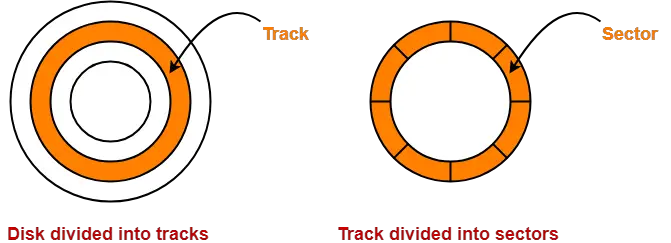• A cylinder is formed by combining the tracks at a given radius of a disk pack.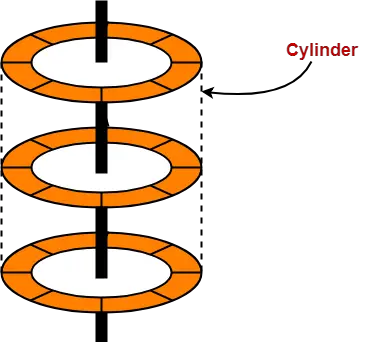• There exists a mechanical arm called as Read / Write head.
• It is used to read from and write to the disk.
• Head has to reach at a particular track and then wait for the rotation of the platter.
• The rotation causes the required sector of the track to come under the head.
• Each platter has 2 surfaces- top and bottom and both the surfaces are used to store the data.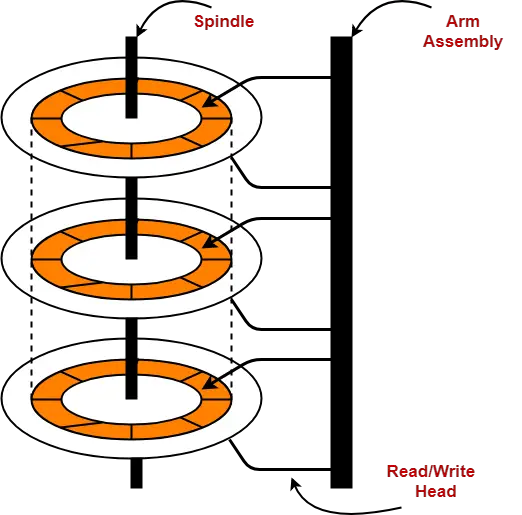## Disk Performance Parameters-

The time taken by the disk to complete an I/O request is called as disk service time or disk access time.

Components that contribute to the service time are-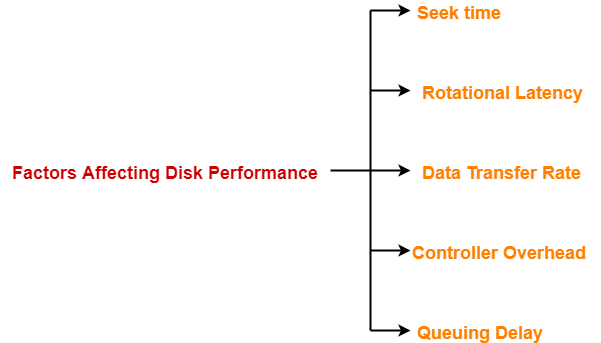1. Seek time
2. Rotational latency
3. Data transfer rate
5. Queuing delay

## 1. Seek Time-

• The time taken by the read / write head to reach the desired track is called as seek time.
• It is the component which contributes the largest percentage of the disk service time.
• The lower the seek time, the faster the I/O operation.

## Specifications

Seek time specifications include-

1. Full stroke
2. Average
3. Track to Track

### 1. Full Stroke-

• It is the time taken by the read / write head to move across the entire width of the disk from the innermost track to the outermost track

### 2. Average-

• It is the average time taken by the read / write head to move from one random track to another.

 Average seek time = 1 / 3 x Full stroke

## 2. Rotational Latency-

• The time taken by the desired sector to come under the read / write head is called as rotational latency.
• It depends on the rotation speed of the spindle.

 Average rotational latency = 1 / 2 x Time taken for full rotation

## 3. Data Transfer Rate-

• The amount of data that passes under the read / write head in a given amount of time is called as data transfer rate.
• The time taken to transfer the data is called as transfer time.

It depends on the following factors-

1. Number of bytes to be transferred
2. Rotation speed of the disk
3. Density of the track
4. Speed of the electronics that connects the disk to the computer

• The overhead imposed by the disk controller is called as controller overhead.
• Disk controller is a device that manages the disk.

## 5. Queuing Delay-

• The time spent waiting for the disk to become free is called as queuing delay.

## NOTE-

 All the tracks of a disk have the same storage capacity.

## Storage Density-

• All the tracks of a disk have the same storage capacity.
• This is because each track has different storage density.
• Storage density decreases as we from one track to another track away from the center.

Thus,

• Innermost track has maximum storage density.
• Outermost track has minimum storage density.

## Important Formulas-

### 1. Disk Access Time-

Disk access time is calculated as-

 Disk access time= Seek time + Rotational delay + Transfer time + Controller overhead + Queuing delay

### 2. Average Disk Access Time-

Average disk access time is calculated as-

 Average disk access time= Average seek time + Average rotational delay + Transfer time + Controller overhead + Queuing delay

### 3. Average Seek Time-

Average seek time is calculated as-

 Average seek time= 1 / 3 x Time taken for one full stroke

Alternatively,

If time taken by the head to move from one track to adjacent track = t units and there are total k tracks, then-

Average seek time

= { Time taken to move from track 1 to track 1 + Time taken to move from track 1 to last track } / 2

= { 0 + (k-1)t } / 2

= (k-1)t / 2

### 4. Average Rotational Latency-

Average rotational latency is calculated as-

 Average rotational latency= 1 / 2 x Time taken for one full rotation

Average rotational latency may also be referred as-

• Average rotational delay
• Average latency
• Average delay

### 5. Capacity Of Disk Pack-

Capacity of a disk pack is calculated as-

 Capacity of a disk pack= Total number of surfaces x Number of tracks per surface x Number of sectors per track x Storage capacity of one sector

### 7. Formatted Disk Space-

Formatted disk space also called as usable disk space is the disk space excluding formatting overhead.

It is calculated as-

 Formatted disk space= Total disk space or capacity – Formatting overhead

### 8. Recording Density Or Storage Density-

Recording density or Storage density is calculated as-

 Storage density of a track= Capacity of the track / Circumference of the track

From here, we can infer-

Storage density of a track ∝ 1 / Circumference of the track

### 9. Track Capacity-

Capacity of a track is calculated as-

 Capacity of a track= Recording density of the track x Circumference of the track

### 10. Data Transfer Rate-

Data transfer rate is calculated as-

 Data transfer rate= Number of heads x Bytes that can be read in one full rotation x Number of rotations in one second

OR

 Data transfer rate= Number of heads x Capacity of one track x Number of rotations in one second

### 11. Tracks Per Surface-

Total number of tracks per surface is calculated as-

 Total number of tracks per surface= (Outer radius – Inner radius) / Inter track gap

## Points to Remember-

• The entire disk space is not usable for storage because some space is wasted in formatting.
• When rotational latency is not given, use average rotational latency for solving numerical problems.
• When seek time is not given, use average seek time for solving numerical problems.
• It is wrong to say that as we move from one track to another away from the center, the capacity increases.
• All the tracks have same storage capacity.

To gain better understanding about magnetic disk-

Watch this Video Lecture

Next Article- Practice Problems On Magnetic Disk

Get more notes and other study material of Computer Organization and Architecture.

Watch video lectures by visiting our YouTube channel LearnVidFun.

## Cache Mapping-

Before you go through this article, make sure that you have gone through the previous article on Cache Mapping.

We have discussed-

• Cache mapping is a technique by which the contents of main memory are brought into the cache.
• Cache mapping is performed using following three different techniques-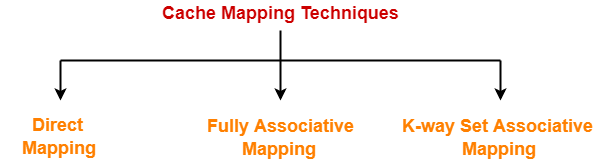In this article, we will discuss practice problems based on cache mapping techniques.

## Problem-01:

The main memory of a computer has 2 cm blocks while the cache has 2c blocks. If the cache uses the set associative mapping scheme with 2 blocks per set, then block k of the main memory maps to the set-

1. (k mod m) of the cache
2. (k mod c) of the cache
3. (k mod 2 c) of the cache
4. (k mod 2 cm) of the cache

## Solution-

Given-

• Number of blocks in main memory = 2 cm
• Number of blocks in cache = 2 c
• Number of blocks in one set of cache = 2

### Number of Sets in Cache-

Number of sets in cache

= Number of blocks in cache / Number of blocks in one set

= 2 c / 2

= c

### Required Mapping-

In set associative mapping,

• Block ‘j’ of main memory maps to set number (j modulo number of sets in cache) of the cache.
• So, block ‘k’ of main memory maps to set number (k mod c) of the cache.
• Thus, option (B) is correct.

Also Read- Practice Problems On Direct Mapping

## Problem-02:

In a k-way set associative cache, the cache is divided into v sets, each of which consists of k lines. The lines of a set placed in sequence one after another. The lines in set s are sequenced before the lines in set (s+1). The main memory blocks are numbered 0 on wards. The main memory block numbered ‘j’ must be mapped to any one of the cache lines from-

1. (j mod v) x k to (j mod v) x k + (k – 1)
2. (j mod v) to (j mod v) + (k – 1)
3. (j mod k) to (j mod k) + (v – 1)
4. (j mod k) x v to (j mod k) x v + (v – 1)

## Solution-

Let-

• 2-way set associative mapping is used, then k = 2
• Number of sets in cache = 4, then v = 4
• Block number ‘3’ has to be mapped, then j = 3

The given options on substituting these values reduce to-

1. (3 mod 4) x 2 to (3 mod 4) x 2 + (2 – 1) = 6 to 7
2. (3 mod 4) to (3 mod 4) + (2 – 1) = 3 to 4
3. (3 mod 2) to (3 mod 2) + (4 – 1) = 1 to 4
4. (3 mod 2) x 4 to (3 mod 2) x 4 + (4 – 1) = 4 to 7

Now, we have been asked to what range of cache lines, block number 3 can be mapped.

According to the above data, the cache memory and main memory will look like-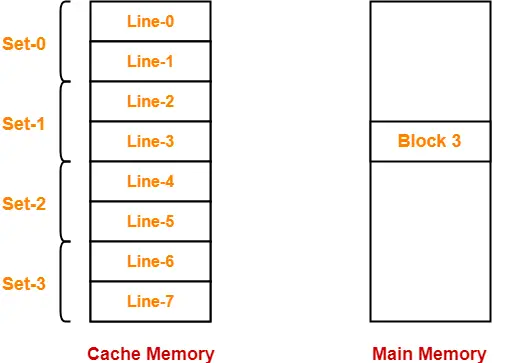In set associative mapping,

• Block ‘j’ of main memory maps to set number (j modulo number of sets in cache) of the cache.
• So, block 3 of main memory maps to set number (3 mod 4) = 3 of cache.
• Within set number 3, block 3 can be mapped to any of the cache lines.
• Thus, block 3 can be mapped to cache lines ranging from 6 to 7.

Thus, Option (A) is correct.

## Problem-03:

A block-set associative cache memory consists of 128 blocks divided into four block sets . The main memory consists of 16,384 blocks and each block contains 256 eight bit words.

1. How many bits are required for addressing the main memory?
2. How many bits are needed to represent the TAG, SET and WORD fields?

## Solution-

Given-

• Number of blocks in cache memory = 128
• Number of blocks in each set of cache = 4
• Main memory size = 16384 blocks
• Block size = 256 bytes
• 1 word = 8 bits = 1 byte

### Main Memory Size-

We have-

Size of main memory

= 16384 blocks

= 16384 x 256 bytes

= 222 bytes

Thus, Number of bits required to address main memory = 22 bits

### Number of Bits in Block Offset-

We have-

Block size

= 256 bytes

= 28 bytes

Thus, Number of bits in block offset or word = 8 bits

### Number of Bits in Set Number-

Number of sets in cache

= Number of lines in cache / Set size

= 128 blocks / 4 blocks

= 32 sets

= 25 sets

Thus, Number of bits in set number = 5 bits

### Number of Bits in Tag Number-

Number of bits in tag

= Number of bits in physical address – (Number of bits in set number + Number of bits in word)

= 22 bits – (5 bits + 8 bits)

= 22 bits – 13 bits

= 9 bits

Thus, Number of bits in tag = 9 bits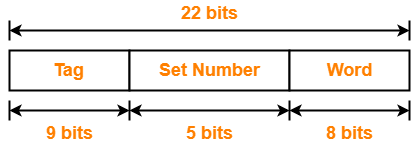## Problem-04:

A computer has a 256 KB, 4-way set associative, write back data cache with block size of 32 bytes. The processor sends 32 bit addresses to the cache controller. Each cache tag directory entry contains in addition to address tag, 2 valid bits, 1 modified bit and 1 replacement bit.

### Part-01:

The number of bits in the tag field of an address is-

1. 11
2. 14
3. 16
4. 27

### Part-02:

The size of the cache tag directory is-

1. 160 Kbits
2. 136 Kbits
3. 40 Kbits
4. 32 Kbits

## Solution-

Given-

• Cache memory size = 256 KB
• Set size = 4 blocks
• Block size = 32 bytes
• Number of bits in physical address = 32 bits

### Number of Bits in Block Offset-

We have-

Block size

= 32 bytes

= 25 bytes

Thus, Number of bits in block offset = 5 bits

### Number of Lines in Cache-

Number of lines in cache

= Cache size / Line size

= 256 KB / 32 bytes

= 218 bytes / 25 bytes

= 213 lines

Thus, Number of lines in cache = 213 lines

### Number of Sets in Cache-

Number of sets in cache

= Number of lines in cache / Set size

= 213 lines / 22 lines

= 211 sets

Thus, Number of bits in set number = 11 bits

### Number of Bits in Tag-

Number of bits in tag

= Number of bits in physical address – (Number of bits in set number + Number of bits in block offset)

= 32 bits – (11 bits + 5 bits)

= 32 bits – 16 bits

= 16 bits

Thus, Number of bits in tag = 16 bits

### Tag Directory Size-

Size of tag directory

= Number of lines in cache x Size of tag

= 213 x (16 bits + 2 valid bits + 1 modified bit + 1 replacement bit)

= 213 x 20 bits

= 163840 bits

= 20 KB or 160 Kbits

Thus,

• For part-01, Option (C) is correct.
• For part-02, Option (A) is correct.

## Problem-05:

A 4-way set associative cache memory unit with a capacity of 16 KB is built using a block size of 8 words. The word length is 32 bits. The size of the physical address space is 4 GB. The number of bits for the TAG field is _____.

## Solution-

Given-

• Set size = 4 lines
• Cache memory size = 16 KB
• Block size = 8 words
• 1 word = 32 bits = 4 bytes
• Main memory size = 4 GB

### Number of Bits in Physical Address-

We have,

Main memory size

= 4 GB

= 232 bytes

Thus, Number of bits in physical address = 32 bits

### Number of Bits in Block Offset-

We have,

Block size

= 8 words

= 8 x 4 bytes

= 32 bytes

= 25 bytes

Thus, Number of bits in block offset = 5 bits

### Number of Lines in Cache-

Number of lines in cache

= Cache size / Line size

= 16 KB / 32 bytes

= 214 bytes / 25 bytes

= 29 lines

= 512 lines

Thus, Number of lines in cache = 512 lines

### Number of Sets in Cache-

Number of sets in cache

= Number of lines in cache / Set size

= 512 lines / 4 lines

= 29 lines / 22 lines

= 27 sets

Thus, Number of bits in set number = 7 bits

### Number of Bits in Tag-

Number of bits in tag

= Number of bits in physical address – (Number of bits in set number + Number of bits in block offset)

= 32 bits – (7 bits + 5 bits)

= 32 bits – 12 bits

= 20 bits

Thus, number of bits in tag = 20 bits

## Problem-06:

If the associativity of a processor cache is doubled while keeping the capacity and block size unchanged, which one of the following is guaranteed to be NOT affected?

1. Width of tag comparator
2. Width of set index decoder
3. Width of way selection multiplexer
4. Width of processor to main memory data bus

## Solution-

Since block size is unchanged, so number of bits in block offset will remain unchanged.

### Effect On Width Of Tag Comparator-

• Associativity of cache is doubled means number of lines in one set is doubled.
• Since number of lines in one set is doubled, therefore number of sets reduces to half.
• Since number of sets reduces to half, so number of bits in set number decrements by 1.
• Since number of bits in set number decrements by 1, so number of bits in tag increments by 1.
• Since number of bits in tag increases, therefore width of tag comparator also increases.

### Effect On Width of Set Index Decoder-

• Associativity of cache is doubled means number of lines in one set is doubled.
• Since number of lines in one set is doubled, therefore number of sets reduces to half.
• Since number of sets reduces to half, so number of bits in set number decrements by 1.
• Since number of bits in set number decreases, therefore width of set index decoder also decreases.

### Effect On Width Of Way Selection Multiplexer-

• Associativity of cache (k) is doubled means number of lines in one set is doubled.
• New associativity of cache is 2k.
• To handle new associativity, size of multiplexers must be 2k x 1.
• Therefore, width of way selection multiplexer increases.

### Effect On Width Of Processor To Main Memory Data Bus-

• Processor to main memory data bus has nothing to do with cache associativity.
• It depends on the number of bits in block offset which is unchanged here.
• So, width of processor to main memory data bus is not affected and remains unchanged.

Thus, Option (D) is correct.

## Problem-07:

Consider a direct mapped cache with 8 cache blocks (0-7). If the memory block requests are in the order-

3, 5, 2, 8, 0, 6, 3, 9, 16, 20, 17, 25, 18, 30, 24, 2, 63, 5, 82, 17, 24

Which of the following memory blocks will not be in the cache at the end of the sequence?

1. 3
2. 18
3. 20
4. 30

Also, calculate the hit ratio and miss ratio.

## Solution-

We have,

• There are 8 blocks in cache memory numbered from 0 to 7.
• In direct mapping, a particular block of main memory is mapped to a particular line of cache memory.
• The line number is given by-

Cache line number = Block address modulo Number of lines in cache

For the given sequence-

• Requests for memory blocks are generated one by one.
• The line number of the block is calculated using the above relation.
• Then, the block is placed in that particular line.
• If already there exists another block in that line, then it is replaced.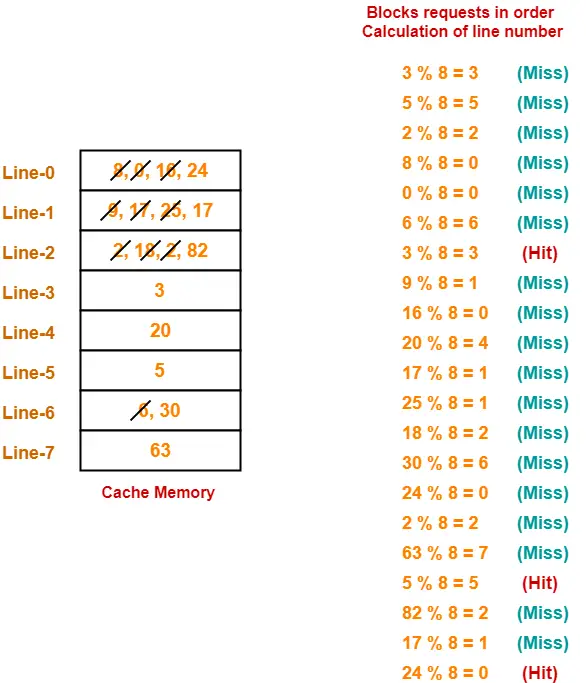Thus,

• Out of given options, only block-18 is not present in the main memory.
• Option-(B) is correct.
• Hit ratio = 3 / 20
• Miss ratio = 17 / 20

## Problem-08:

Consider a fully associative cache with 8 cache blocks (0-7). The memory block requests are in the order-

4, 3, 25, 8, 19, 6, 25, 8, 16, 35, 45, 22, 8, 3, 16, 25, 7

If LRU replacement policy is used, which cache block will have memory block 7?

Also, calculate the hit ratio and miss ratio.

## Solution-

We have,

• There are 8 blocks in cache memory numbered from 0 to 7.
• In fully associative mapping, any block of main memory can be mapped to any line of the cache that is freely available.
• If all the cache lines are already occupied, then a block is replaced in accordance with the replacement policy.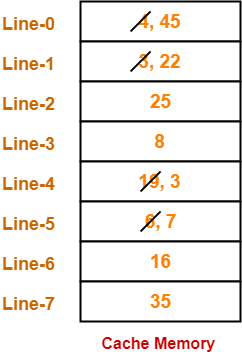Thus,

• Line-5 contains the block-7.
• Hit ratio = 5 / 17
• Miss ratio = 12 / 17

## Problem-09:

Consider a 4-way set associative mapping with 16 cache blocks. The memory block requests are in the order-

0, 255, 1, 4, 3, 8, 133, 159, 216, 129, 63, 8, 48, 32, 73, 92, 155

If LRU replacement policy is used, which cache block will not be present in the cache?

1. 3
2. 8
3. 129
4. 216

Also, calculate the hit ratio and miss ratio.

## Solution-

We have,

• There are 16 blocks in cache memory numbered from 0 to 15.
• Each set contains 4 cache lines.
• In set associative mapping, a particular block of main memory is mapped to a particular set of cache memory.
• The set number is given by-

Cache line number = Block address modulo Number of sets in cache

For the given sequence-

• Requests for memory blocks are generated one by one.
• The set number of the block is calculated using the above relation.
• Within that set, the block is placed in any freely available cache line.
• If all the blocks are already occupied, then one of the block is replaced in accordance with the employed replacement policy.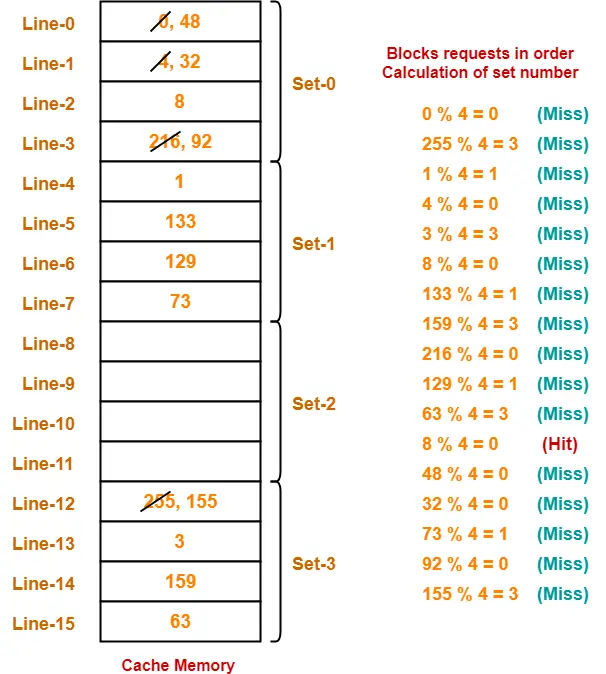Thus,

• Out of given options, only block-216 is not present in the main memory.
• Option-(D) is correct.
• Hit ratio = 1 / 17
• Miss ratio = 16 / 17

## Problem-10:

Consider a small 2-way set associative mapping with a total of 4 blocks. LRU replacement policy is used for choosing the block to be replaced. The number of cache misses for the following sequence of block addresses 8, 12, 0, 12, 8 is ____.

## Solution-

• Practice yourself.
• Total number of cache misses = 4

## Problem-11:

Consider the cache has 4 blocks. For the memory references-

5, 12, 13, 17, 4, 12, 13, 17, 2, 13, 19, 13, 43, 61, 19

What is the hit ratio for the following cache replacement algorithms-

1. FIFO
2. LRU
3. Direct mapping
4. 2-way set associative mapping using LRU

## Solution-

• Practice yourself.
• Using FIFO as cache replacement algorithm, hit ratio = 5/15 = 1/3.
• Using LRU as cache replacement algorithm, hit ratio = 6/15 = 2/5.
• Using direct mapping as cache replacement algorithm, hit ratio = 1/15.
• Using 2-way set associative mapping as cache replacement algorithm, hit ratio = 5/15 = 1/3

## Problem-12:

A hierarchical memory system has the following specification, 20 MB main storage with access time of 300 ns, 256 bytes cache with access time of 50 ns, word size 4 bytes, page size 8 words. What will be the hit ratio if the page address trace of a program has the pattern 0, 1, 2, 3, 0, 1, 2, 4 following LRU page replacement technique?

## Solution-

Given-

• Main memory size = 20 MB
• Main memory access time = 300 ns
• Cache memory size = 256 bytes
• Cache memory access time = 50 ns
• Word size = 4 bytes
• Page size = 8 words

We have,

Line size

= 8 words

= 8 x 4 bytes

= 32 bytes

### Number of Lines in Cache-

Number of lines in cache

= Cache size / Line size

= 256 bytes / 32 bytes

= 8 lines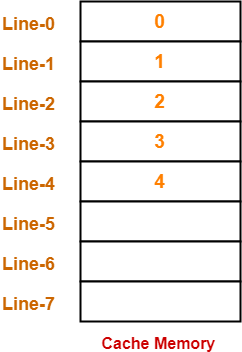Thus,

• Number of hits = 3
• Hit ratio = 3 / 8

## Problem-13:

Consider an array A and each element occupies 4 words. A 32 word cache is used and divided into 8 word blocks. What is the hit ratio for the following code-

for (i=0 ; i < 100 ; i++)

A[i] = A[i] + 10;

## Solution-

Number of lines in cache

= Cache size / Line size

= 32 words / 8 words

= 4 lines

Since each element of the array occupies 4 words, so-

Number of elements that can be placed in one line = 2

Now, let us analyze the statement-

A[i] = A[i] + 10;

For each i,

• Firstly, the value of A[i] is read.
• Secondly, 10 is added to the A[i].
• Thirdly, the updated value of A[i] is written back.

Thus,

• For each i, A[i] is accessed two times.
• Two memory accesses are required-one for read operation and other for write operation.

Assume the cache is all empty initially.

In the first loop iteration,

• First, value of A has to be read.
• Since cache is empty, so A is brought in the cache.
• Along with A, element A also enters the cache since each block can hold 2 elements of the array.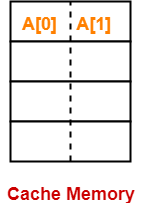• Thus, For A, a miss occurred for the read operation.
• Now, 10 is added to the value of A.
• Now, the updated value has to be written back to A.
• Again cache memory is accessed to get A for writing its updated value.
• This time, for A, a hit occurs for the write operation.

In the second loop iteration,

• First, value of A has to be read.
• A is already present in the cache.
• Thus, For A. a hit occurs for the read operation.
• Now, 10 is added to the value of A.
• Now, the updated value has to be written back to A.
• Again cache memory is accessed to get A for writing its updated value.
• Again, for A, a hit occurs for the write operation.

In the similar manner, for every next two consecutive elements-

• There will be a miss for the read operation for the first element.
• There will be a hit for the write operation for the first element.
• There will be a hit for both read and write operations for the second element.

Likewise, for 100 elements, we will have 50 such pairs in cache and in every pair, there will be one miss and three hits.

Thus,

• Total number of hits = 50 x 3 = 150
• Total number of misses = 50 x 1 = 50
• Total number of references = 200 (100 for read and 100 for write)

Thus,

• Hit ratio = 150 / 200 = 3 / 4 = 0.75
• Miss ratio = 50 / 200 = 1 / 4 = 0.25

## Problem-14:

Consider an array has 100 elements and each element occupies 4 words. A 32 bit word cache is used and divided into a block of 8 words.

What is the hit ratio for the following statement-

for (i=0 ; i < 10 ; i++)

for (j=0 ; j < 10 ; j++)

A[i][j] = A[i][j] + 10;

if-

1. Row major order is used
2. Column major order is used

## Solution-

According to question, cache is divided as-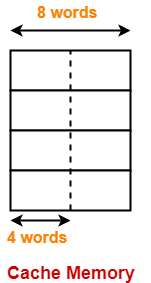In each cache line, two elements of the array can reside.

### Case-01: When Row Major Order is Used-

In row major order, elements of the array are stored row wise in the memory as-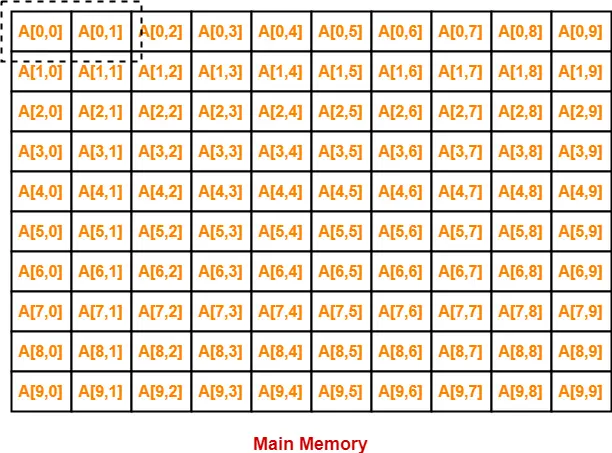• In the first iteration, when A will be brought in the cache, A will also be brought.
• Then, things goes as it went in the previous question.
• There will be a miss for A[0,0] read operation and hit for A[0,0] write operation.
• For A[0,1], there will be a hit for both read and write operations.
• Similar will be the story with every next two consecutive elements.

Thus,

• Total number of hits = 50 x 3 = 150
• Total number of misses = 50 x 1 = 50
• Total number of references = 200 (100 for read and 100 for write)

Thus,

• Hit ratio = 150 / 200 = 3 / 4 = 0.75
• Miss ratio = 50 / 200 = 1 / 4 = 0.25

### Case-02: When Column Major Order is Used-

In column major order, elements of the array are stored column wise in the memory as-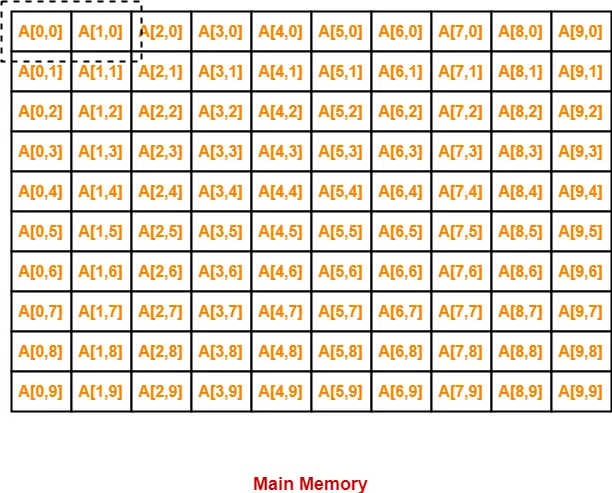• In the first iteration, when A[0,0] will be brought in the cache, A will also be brought.
• This time A will not be useful in iteration-2.
• A will be needed after surpassing 10 element.
• But by that time, this block would have got replaced since there are only 4 lines in the cache.
• For A to be useful, there has to be at least 10 lines in the cache.

Thus,

Under given scenario, for each element of the array-

• There will be a miss for the read operation.
• There will be a hit for the write operation.

Thus,

• Total number of hits = 100 x 1 = 100
• Total number of misses = 100 x 1 = 100
• Total number of references = 200 (100 for read and 100 for write)

Thus,

• Hit ratio = 100 / 200 = 1 / 2 = 0.50
• Miss ratio = 100 / 200 = 1 / 2 = 0.50

To increase the hit rate, following measures can be taken-

• Row major order can be used (Hit rate = 75%)
• The statement A[i][j] = A[i][j] + 10 can be replaced with A[j,i] = A[j][i] + 10 (Hit rate = 75%)

## Problem-15:

An access sequence of cache block addresses is of length N and contains n unique block addresses. The number of unique block addresses between two consecutive accesses to the same block address is bounded above by k. What is the miss ratio if the access sequence is passed through a cache of associativity A>=k exercising LRU replacement policy?

1. n/N
2. 1/N
3. 1/A
4. k/n

## Solution-

Required miss ratio = n/N.

Thus, Option (A) is correct.

Next Article- Cache Line | Effects of Changing Cache Line Size

Get more notes and other study material of Computer Organization and Architecture.

Watch video lectures by visiting our YouTube channel LearnVidFun.